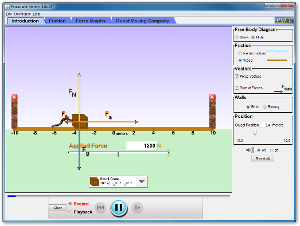Physics Core/AP 1 and 2

#### Course Materials are always under revision! Weblecture content may change anytime prior to two weeks before scheduled chat session for content.Homework

# Physics Chapter 4: 5-7: Newton's Third Law of Motion and Weight

## Homework

### Reading Preparation

Text Reading: Giancoli, Physics - Principles with Applications, Chapter 4: Sections 5-7

##### Study Points
• Section 5: : Newton's third law of motion is trickier: but it means that if object A exerts a force on a solid object B, object B pushes back with the same magnitude, but in the opposite direction. This makes walking possible.
• Section 6: If the weight of an object is not met by an equal and opposite force, the object will fall downward. If the object is restrained (by a shelf or a floor), the equal and opposite force exerted is the normal force, where normal means perpendicular to the surface exerting the force.
• Section 7: If the weight of an object and the normal force of its restraining surface are not equal and opposite, then a net force will result, one which accelerates the object (which no longer remains motionless). We can draw diagrams that help us determine the interplay of forces acting on an object, and their net result. Among the practical applications we need to understand using this type of analysis are objects on slopes and pulleys.

### Key Equations

Gravitational force:

Normal force (note that if the angle is 90°, cos θ = 1 and normal force is equal but opposite to gravitaitonal force):

Force due to friction:

### Web Lecture

Read the following weblecture before chat: Vector Forces and Newton's Laws

### Study Activity

The website below will either download a Java applet to your computer or run the applet in place, depending on how your browser is configured. You will need Java installed to run the applet locally on your computer.

The "friction" simulation will download to a java program, which you may be able to run if you have Java libraries installed on your computer.Click to Run

Explore the forces at work when you try to push a filing cabinet. Create an applied force and see the resulting friction force and total force acting on the cabinet. Charts show the forces, position, velocity, and acceleration vs. time. View a Free Body Diagram of all the forces (including gravitational and normal forces).

Physics simulation Java Applets are the product of the PHET Interactive Simulations project at the University of Colorado, Boulder.

### Chat Preparation Activities

• Forum question: The Moodle forum for the session will assign a specific study question for you to prepare for chat. You need to read this question and post your answer before chat starts for this session.
• Mastery Exercise: The Moodle Mastery exercise for the chapter will contain sections related to our chat topic. Try to complete these before the chat starts, so that you can ask questions.

### Chapter Quiz

• The chapter quiz is not yet due.

### Lab Work

If you want lab credit for this course, you must complete at least 12 labs (honors course) or 18 labs (AP students). One or more lab exercises are posted for each chapter as part of the homework assignment. We will be reviewing lab work at regular intervals, so do not get behind!